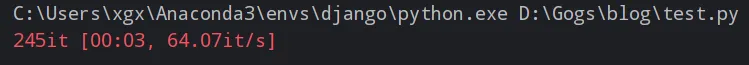欢迎访问我的网站，希望内容对您有用，感兴趣的可以加入免费知识星球。

# Python进度条显示方案

3周前 (09-09) 134次浏览

## 简介

`tqdm` 是一个非常常用的 `Python` 进度条库，它可以在循环迭代和 `IO` 操作期间添加一个进度条，直观地显示循环迭代的进程。

`tqdm` 是在2013年发布的，目的是为 `Python` 提供一个简单、轻量级的进度条解决方案。随后 `tqdm` 又增加了对 `Jupyter notebook`的支持，也支持在 `pandas``PySpark` 等数据处理操作中使用。现在 `tqdm` 已经被广泛应用于机器学习、深度学习等 `Python` 项目中。

`tqdm` 使用迭代器来封装你的迭代对象，追踪迭代次数，并在每次迭代时打印进度条。实际上它会在一个线程内对迭代器进行监控，不会影响原迭代的性能。

## 安装

``pip install tqdm``

## 示例代码

``````import time
from tqdm import tqdm

# 简单的for循环
for i in tqdm(range(1000)):
time.sleep(0.01)````````````import time
from tqdm import trange

for i in trange(1000):
time.sleep(0.01)```````tqdm` 传入数组

``````import time
from tqdm import tqdm

for char in tqdm(["a", "b", "c", "d", "e", "f", "g"]):
time.sleep(0.01)``````

``````import time
from tqdm import tqdm

# 读取文件中的数据
with open('file.txt') as f:
for line in tqdm(f):
time.sleep(0.01)```````pandas` 中使用

``````import pandas as pd
import numpy as np
from tqdm import tqdm

df = pd.DataFrame(np.random.randint(0, 500, (100000, 6)))
tqdm.pandas(desc="Demo")
df.progress_apply(lambda x: x**2)``````